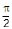# Test: Tangent And Normal (Competition Level) - 2

## 9 Questions MCQ Test Mathematics (Maths) Class 12 | Test: Tangent And Normal (Competition Level) - 2

Description
This mock test of Test: Tangent And Normal (Competition Level) - 2 for JEE helps you for every JEE entrance exam. This contains 9 Multiple Choice Questions for JEE Test: Tangent And Normal (Competition Level) - 2 (mcq) to study with solutions a complete question bank. The solved questions answers in this Test: Tangent And Normal (Competition Level) - 2 quiz give you a good mix of easy questions and tough questions. JEE students definitely take this Test: Tangent And Normal (Competition Level) - 2 exercise for a better result in the exam. You can find other Test: Tangent And Normal (Competition Level) - 2 extra questions, long questions & short questions for JEE on EduRev as well by searching above.
QUESTION: 1

Solution:
QUESTION: 2

Solution:
QUESTION: 3

### If the subnormal at any point on y = a1 – n xn is of constant length, then the value of n is

Solution:
QUESTION: 4

If the tangent at P of the curve y2 = x3 intersects the curve again at Q and the straight lines OP, OQ make angles a, b with the x–axis, where `O' is the origin, then tan a/tan b has the value equal to

Solution:
QUESTION: 5

The length of the normal to the curve x = a(q + sin q), y = a (1 – cos q), at q =is

Solution:
QUESTION: 6

The beds of two rivers (within a certain region) are a parabola y = x2 and a straight line y = x – 2. These rivers are to be connected by a straight canal. The co-ordinates of the ends of the shortest canal can be

Solution:
QUESTION: 7

If the area of the triangle included between the axes and any tangent to the curve xn y = an is constant, then n is equal to

Solution:
QUESTION: 8

At (0, 0), the curve y2 = x3 + x2

Solution:
QUESTION: 9

For the curve x = t2 – 1, y = t2 – t, the tangent line is perpendicular to x-axis where

Solution:

Given curve is x = t− 1,y = t− t
Derivating w.r.to t we get
d x/ dt =2t -------(1)
and  dy / dt = 2t − 1 -------(2)
dividing (2) by (1)  we get
dy / dx = (2t − 1) / 2t
Therefore, the slope of the tangent is  (2t − 1) / 2t
Given that the tangent is perpendicular to x-axis. Therefore, tangent is parallel to y-axis.
We know that slope of y-axis is infinity and the slopes of the two parallel lines are equal.
Therefore, slope of the tangent is infinity.
Hence, (2t − 1) / 2t = 1/0
⟹ 2t = 0
⟹ t = 0